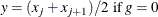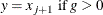### Percentile Definitions

You can use the PCTLDEF= option to specify one of five definitions for computing quantile statistics (percentiles). Suppose that n is the number of nonmissing values for a variable and that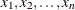represent the ordered values of the analysis variable. For the tth percentile, set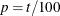.

For the following definitions numbered 1, 2, 3, and 5, express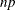as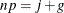where j is the integer part of, and g is the fractional part of. For definition 4, let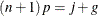The tth percentile (call it y) can be defined as follows:

PCTLDEF=1

weighted average at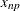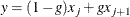where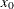is taken to be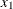.

PCTLDEF=2

observation numbered closest to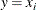where i is the integer part of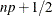if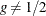. If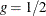, then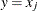if j is even, or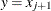if j is odd.

PCTLDEF=3

empirical distribution function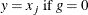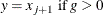PCTLDEF=4

weighted average aimed at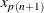where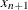is taken to be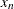.

PCTLDEF=5

empirical distribution function with averaging